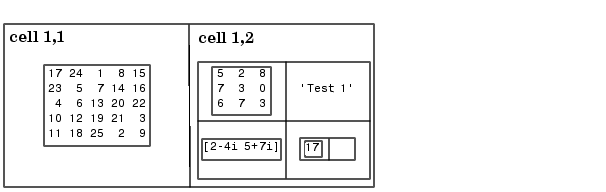ProgrammingNesting Cell Arrays

A cell can contain another cell array, or even an array of cell arrays. (Cells that contain noncell data are called leaf cells.) You can use nested curly braces, the `cell` function, or direct assignment statements to create nested cell arrays. You can then access and manipulate individual cells, subarrays of cells, or cell elements.

Building Nested Arrays with Nested Curly Braces

You can nest pairs of curly braces to create a nested cell array. For example,

• ```clear A
A(1,1) = {magic(5)};

A(1,2) = {{[5 2 8; 7 3 0; 6 7 3] 'Test 1'; [2-4i 5+7i] {17 []}}}
A =
[5x5 double]    {2x2 cell}
```

Note that the right side of the assignment is enclosed in two sets of curly braces. The first set represents cell `(1,2)` of cell array `A`. The second "packages" the 2-by-2 cell array inside the outer cell.

Building Nested Arrays with the cell Function

To nest cell arrays with the `cell` function, assign the output of `cell` to an existing cell:

1. Create an empty 1-by-2 cell array.
• ```A = cell(1,2);
```
2. Create a 2-by-2 cell array inside `A(1,2)`.
• ```A(1,2) = {cell(2,2)};
```
3. Fill `A`, including the nested array, using assignments.
• ```A(1,1) = {magic(5)};
A{1,2}(1,1) = {[5 2 8; 7 3 0; 6 7 3]};
A{1,2}(1,2) = {'Test 1'};
A{1,2}(2,1) = {[2-4i 5+7i]};
A{1,2}(2,2) = {cell(1, 2)}
A{1,2}{2,2}(1) = {17};
```
1. Note the use of curly braces until the final level of nested subscripts. This is required because you need to access cell contents to access cells within cells.

You can also build nested cell arrays with direct assignments using the statements shown in step 3 above.

Indexing Nested Cell Arrays

To index nested cells, concatenate indexing expressions. The first set of subscripts accesses the top layer of cells, and subsequent sets of parentheses access successively deeper layers.

For example, array `A` has three levels of nesting:• To access the 5-by-5 array in cell `(1,1)`, use `A{1,1}`.
• To access the 3-by-3 array in position `(1,1)` of cell `(1,2)`, use `A{1,2}{1,1}`.
• To access the 2-by-2 cell array in cell `(1,2)`, use `A{1,2}`.
• To access the empty cell in position `(2,2)` of cell `(1,2)`, use `A{1,2}{2,2}{1,2}`.Organizing Data in Cell Arrays Converting Between Cell and Numeric Arrays© 1994-2005 The MathWorks, Inc.